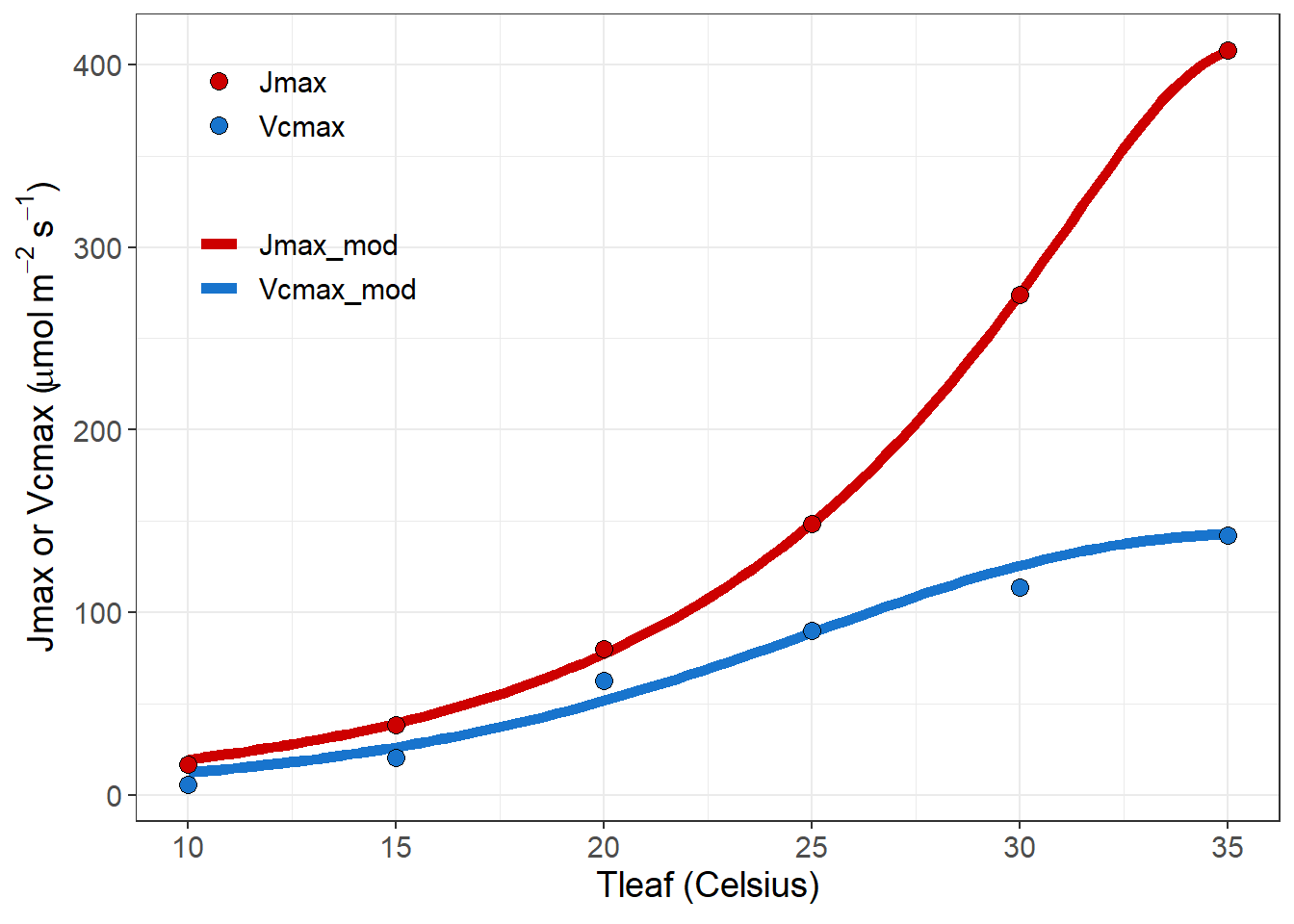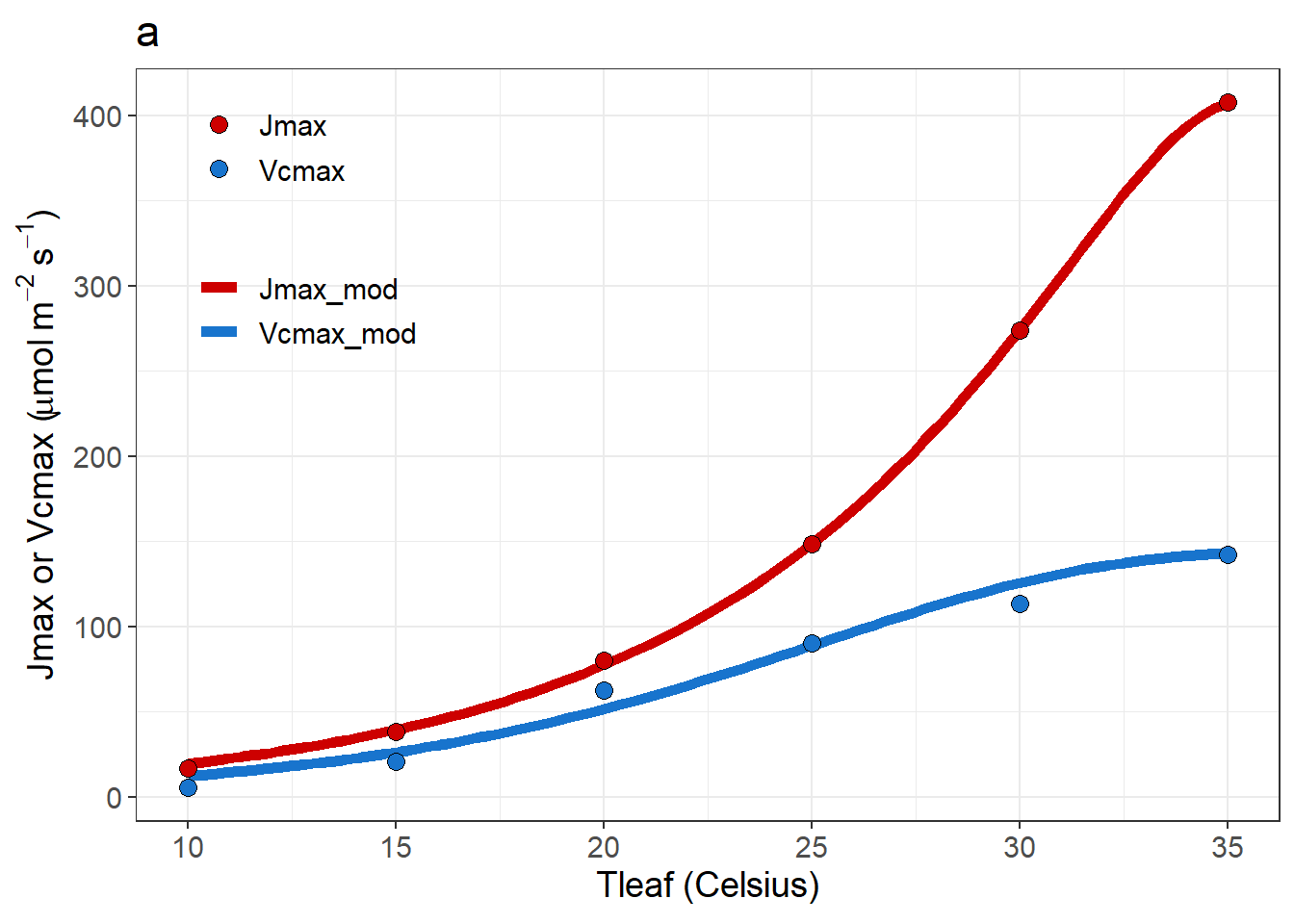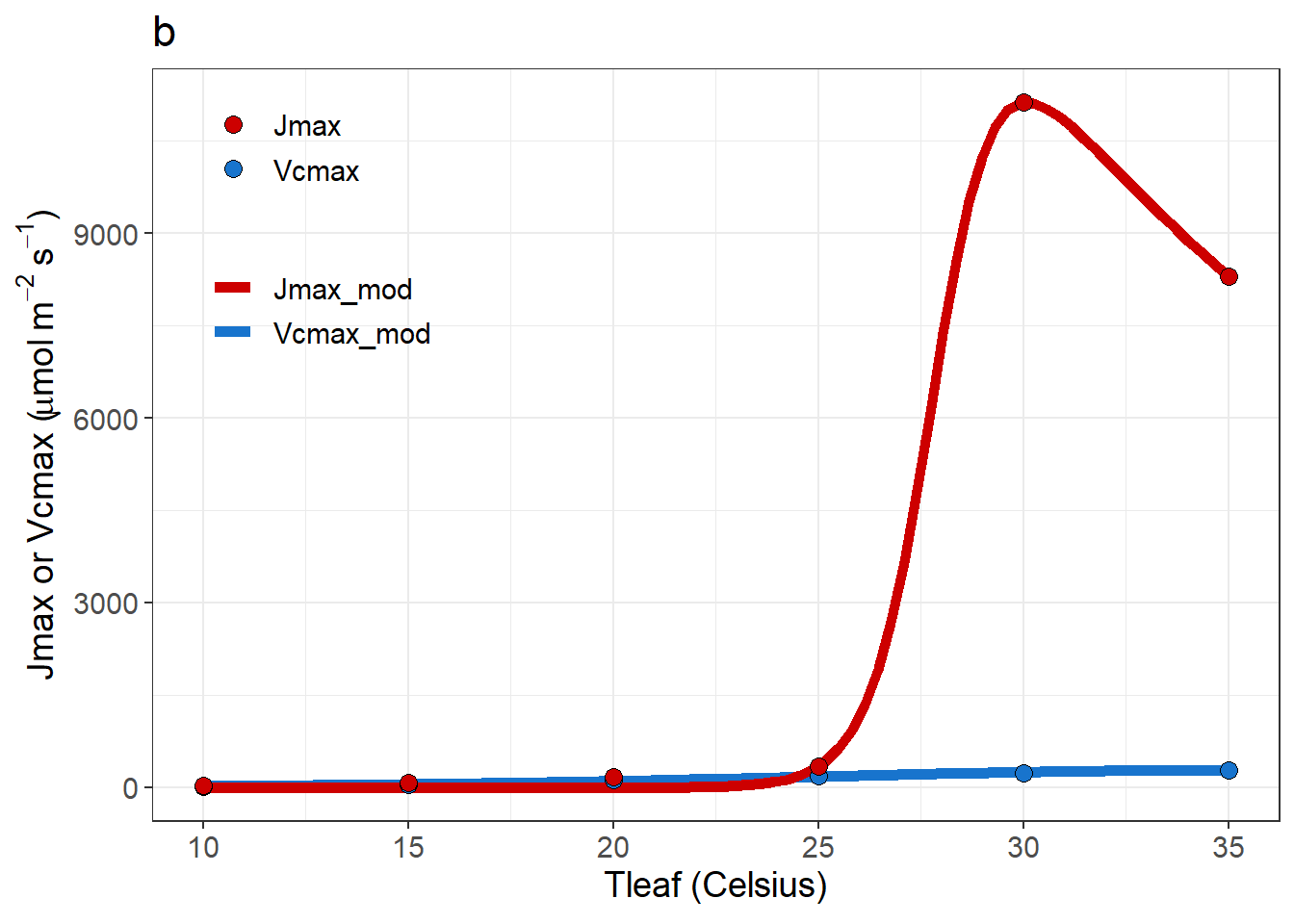library(plantecowrap)

Fit ACi Curves then fit temperature responses

#Read in data from package
data <- read.csv(system.file("extdata", "example_1.csv",
package = "plantecowrap"),
stringsAsFactors = FALSE)
#Fit ACi curves
fits <- fitacis2(data = data,
varnames = list(ALEAF = "A",
Tleaf = "Tleaf",
Ci = "Ci",
PPFD = "PPFD",
Rd = "Rd",
Press = "Press"),
group1 = "Treat",
fitTPU = FALSE,
fitmethod = "bilinear",
gm25 = 10000, #Allows fitting on an ACi rather than ACc-basis
Egm = 0)

#Get ACi outputs
outputs <- acisummary(data, group1 = "Treat", fits = fits)

#Fit temperature response
#Note that this will return NA warnings because it runs through
#1000 sets of starting parameters for Vcmax and Jmax
tresp <- fit_topt_VJ(outputs)
## Warning in fit_topt_VJ(outputs): NAs introduced by coercion

## Warning in fit_topt_VJ(outputs): NAs introduced by coercion

## Warning in fit_topt_VJ(outputs): NAs introduced by coercion

## Warning in fit_topt_VJ(outputs): NAs introduced by coercion

## Warning in fit_topt_VJ(outputs): NAs introduced by coercion

## Warning in fit_topt_VJ(outputs): NAs introduced by coercion
## Warning in nls.lm(par = start, fn = FCT, jac = jac, control = control, lower = lower, : lmdif: info = -1. Number of iterations has reached maxiter' == 100.
## Warning in fit_topt_VJ(outputs): NAs introduced by coercion

## Warning in fit_topt_VJ(outputs): NAs introduced by coercion

## Warning in fit_topt_VJ(outputs): NAs introduced by coercion

## Warning in fit_topt_VJ(outputs): NAs introduced by coercion

## Warning in fit_topt_VJ(outputs): NAs introduced by coercion

## Warning in fit_topt_VJ(outputs): NAs introduced by coercion
#See parameters
tresp[]
##   Parameter      k25       Ea Residual      BIC
## 1     Vcmax  75.1461 53.45129 73.99293 53.18597
## 2      Jmax 148.6158 78.94620 60.95280 52.36863
#See plot
tresp[]#Fitting of multiple temperature responses

#Read in data from package
data2 <- read.csv(system.file("extdata", "example_2.csv",
package = "plantecowrap"),
stringsAsFactors = FALSE)
#Fit ACi curves
fits2 <- fitacis2(data = data2,
varnames = list(ALEAF = "A",
Tleaf = "Tleaf",
Ci = "Ci",
PPFD = "PPFD",
Rd = "Rd",
Press = "Press"),
group1 = "Grouping",
fitTPU = FALSE,
fitmethod = "bilinear",
gm25 = 10000,
Egm = 0)

#Get ACi outputs
outputs <- acisummary(data2, group1 = "Grouping", fits = fits2)
#NOTE: if fitacis2 fails to fit a curve, some output elements will be "Failed"
#and need to be removed before acisummary() is run

#Split grouping variable
outputs <- separate(outputs, col = "ID", into = c("Treat", "Block"), sep = "_")

#Note that this will return NA warnings because it runs through
#1000 sets of starting parameters for Vcmax and Jmax. Some of
#these sets will not work and return the NA warning.
tresps <- fit_topt_VJs(data = outputs,
group = "Block")
## Warning in fit_topt_VJ(data = data[[i]], varnames = varnames, title =
## names(data[i]), : NAs introduced by coercion

## Warning in fit_topt_VJ(data = data[[i]], varnames = varnames, title =
## names(data[i]), : NAs introduced by coercion

## Warning in fit_topt_VJ(data = data[[i]], varnames = varnames, title =
## names(data[i]), : NAs introduced by coercion

## Warning in fit_topt_VJ(data = data[[i]], varnames = varnames, title =
## names(data[i]), : NAs introduced by coercion

## Warning in fit_topt_VJ(data = data[[i]], varnames = varnames, title =
## names(data[i]), : NAs introduced by coercion

## Warning in fit_topt_VJ(data = data[[i]], varnames = varnames, title =
## names(data[i]), : NAs introduced by coercion
## Warning in nls.lm(par = start, fn = FCT, jac = jac, control = control, lower = lower, : lmdif: info = -1. Number of iterations has reached maxiter' == 100.
## Warning in fit_topt_VJ(data = data[[i]], varnames = varnames, title =
## names(data[i]), : NAs introduced by coercion

## Warning in fit_topt_VJ(data = data[[i]], varnames = varnames, title =
## names(data[i]), : NAs introduced by coercion

## Warning in fit_topt_VJ(data = data[[i]], varnames = varnames, title =
## names(data[i]), : NAs introduced by coercion

## Warning in fit_topt_VJ(data = data[[i]], varnames = varnames, title =
## names(data[i]), : NAs introduced by coercion

## Warning in fit_topt_VJ(data = data[[i]], varnames = varnames, title =
## names(data[i]), : NAs introduced by coercion

## Warning in fit_topt_VJ(data = data[[i]], varnames = varnames, title =
## names(data[i]), : NAs introduced by coercion

## Warning in fit_topt_VJ(data = data[[i]], varnames = varnames, title =
## names(data[i]), : NAs introduced by coercion

## Warning in fit_topt_VJ(data = data[[i]], varnames = varnames, title =
## names(data[i]), : NAs introduced by coercion

## Warning in fit_topt_VJ(data = data[[i]], varnames = varnames, title =
## names(data[i]), : NAs introduced by coercion

## Warning in fit_topt_VJ(data = data[[i]], varnames = varnames, title =
## names(data[i]), : NAs introduced by coercion

## Warning in fit_topt_VJ(data = data[[i]], varnames = varnames, title =
## names(data[i]), : NAs introduced by coercion

## Warning in fit_topt_VJ(data = data[[i]], varnames = varnames, title =
## names(data[i]), : NAs introduced by coercion

## Warning in fit_topt_VJ(data = data[[i]], varnames = varnames, title =
## names(data[i]), : NAs introduced by coercion

## Warning in fit_topt_VJ(data = data[[i]], varnames = varnames, title =
## names(data[i]), : NAs introduced by coercion

## Warning in fit_topt_VJ(data = data[[i]], varnames = varnames, title =
## names(data[i]), : NAs introduced by coercion

## Warning in fit_topt_VJ(data = data[[i]], varnames = varnames, title =
## names(data[i]), : NAs introduced by coercion

## Warning in fit_topt_VJ(data = data[[i]], varnames = varnames, title =
## names(data[i]), : NAs introduced by coercion

## Warning in fit_topt_VJ(data = data[[i]], varnames = varnames, title =
## names(data[i]), : NAs introduced by coercion
#Get parameters
pars <- get_t_pars(tresps)

#Get graphs
graphs <- get_t_graphs(tresps)

#Print graphs
#To print to current working directory, set path = "./".
print_graphs(graphs,
path = tempdir())
## $a ## ##$b
#View parameters
pars
##            Ea       Hd       kopt     Topt   residual      BIC Parameter ID
## 826 106.75701 142.7305   143.3115 36.00000  37.035038 50.24750     Vcmax  a
## 635  95.19201 382.7120   408.6462 35.41034   7.328159 31.74101      Jmax  a
## 657 107.91985 142.5541   285.6264 36.00000  71.942181 58.42544     Vcmax  b
## 679 942.06739 997.3328 11123.9346 30.07649 279.263391 77.94101      Jmax  b
#View graphs
#Note that the second graph is strange because A was simply multiplied by
#2 for the sample dataset
graphs[]
## $agraphs[] ##$b